# Chemistry Sample Paper For Class 12 With Solution NCERT CBSE Board 2020

Here we are providing CBSE Previous Year Question Papers Class 6 to 12 solved with soutions Chemistry Sample Paper for Class 12 With Solution NCERT CBSE Board 2020 Chemistry sample paper class 12, Chemistry previous year question paper class 12, cbse class 12 Chemistry sample paper, cbse class 12 Chemistry sample paper 2020, Chemistry sample paper class 12 2020, cbse sample paper 2020 class 12 Chemistry, class Practice of previous year question papers and sample papers protects each and every student to score bad marks in exams.If any student of CBSE Board continuously practices last year question paper student will easily score high marks in tests. Fortunately earlier year question papers can assist the understudies with scoring great in the tests. Unraveling previous year question paper class 12 Chemistry is significant for understudies who will show up for Class 12 Board tests.

## Class 12 Subject Chemistry Paper Set 4 with Solutions

SECTION – A

Read the given passage and answer the questions 1 to 5 that follow :

Colloidal particles always carry an electric charge which may be either positive or negative. For example, when AgNO3 solution is added to KI solution, a negatively charged colloidal sol is obtained. The presence of equal and similar charges on colloidal particles provide stability to the colloidal sol and if, somehow, charge is removed, coagulation of sol occurs. Lyophobic sols are readily coagulated as compare to lyophilic sols.

Question 1: (Marks 1)

What is the reason for the charge on sol particles ?

Answer :

Due to preferential adsorption of common ions from solution / due to electron capture by sol particles during electrodispersion of metal/ due to formulation of electrical double layer

Question 2: (Marks 1)

Why the presence of equal and similar charges on colloidal particles provide stability ?

Answer :

Due to repulsion between the particles of similar charge.

Question 3: (Marks 1)

Why a negatively charged sol is obtained on adding AgNO3 solution to KI solution ?

Answer :

Due to preferential adsorption of I from dispersion medium

Question 4: (Marks 1)

Name one method by which coagulation of lyophobic sol can be carried out.

Answer :

By electrophoresis / by mixing two oppositely charged sols / by boiling / by persistent dialysis / by addition of electrolyte.

Question 5: (Marks 1)

Out of KI or K2SO4 , which electrolyte is better in the coagulation of positive sol ?

Answer :

K2SO4

Questions 6 to 10 are one word answers :

Question 6: (Marks 1)

Name the method applied for the concentration of Bauxite ore in the extraction of Aluminium.

Answer :

Leaching / Baeyer’s process

Question 7: (Marks 1)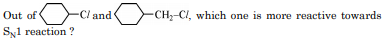Answer :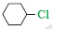Question 8: (Marks 1)

Write an isomer of C3H9N which gives foul smell of isocyanide when treated with chloroform and ethanolic NaOH.

Answer :

CH3–CH2–CH2–NH2

Question 9: (Marks 1)

Which one of the following is an antidepressant drug ? Chloramphenicol, Luminal, Bithional

Answer :

Luminal

Question 10: (Marks 1)

Write the name of component of starch which is water soluble.

Answer :

Amylose

Questions 11 to 15 are Multiple Choice Questions :

Question 11: (Marks 1)

How many ions are produced from the complex [Co(NH3 )5Cl] Cl2 in solution ?

(a) 4

(b) 2

(c) 3

(d) 5

Answer :

(c) 3

Question 12: (Marks 1)

In a lead storage battery

(a) PbO2 is reduced to PbSO4 at the cathode.

(b) Pb is oxidised to PbSO4 at the anode.

(c) Both electrodes are immersed in the same aqueous solution of H2SO4 .

(d) All the above are true.

Answer :

(d) All the above are true.

Question 13: (Marks 1)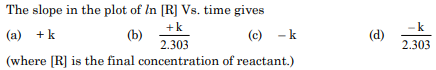Answer :

(c) – k

Question 14: (Marks 1)

The pair [Co(NH3)4Cl2] Br2 and [Co(NH3)4 Br2] Cl2 will show

(a) Linkage isomerism

(b) Hydrate isomerism

(c) Ionization isomerism

(d) Coordinate isomerism

Answer :

(c) Ionization isomerism

Question 15: (Marks 1)

An α-helix is a structural feature of

(a) Sucrose

(b) Polypeptides

(c) Nucleotides

(d) Starch

Answer :

(c) Nucleotides

Questions 16 to 20 :

(A) Both Assertion (A) and Reason (R) are correct statements, and Reason (R) is the correct explanation of the Assertion (A).

(B) Both Assertion (A) and Reason (R) are correct statements, but Reason (R) is not the correct explanation of the Assertion (A).

(C) Assertion (A) is correct, but Reason (R) is wrong statement.

(D) Assertion (A) is wrong, but Reason (R) is correct statement.

Question 16: (Marks 1)

Assertion (A) : F2 is a strong oxidising agent.

Reason (R) : Electron gain enthalpy of fluorine is less negative.

Answer :

(B) Both Assertion (A) and Reason (R) are correct statements, but Reason (R) is not the correct explanation of the Assertion (A).

Question 17: (Marks 1)

Assertion (A) : (CH3)3 C–O–CH3 gives (CH3)3 C–I and CH3OH on treatment with HI.

Reason (R) : The reaction occurs by SN1 mechanism.

Answer :

(A) Both Assertion (A) and Reason (R) are correct statements, and Reason (R) is the correct explanation of the Assertion (A).

Question 18: (Marks 1)

Assertion (A) : Transition metals have low melting points.

Reason (R) : The involvement of greater number of (n – 1)d and ns electrons in the interatomic metallic bonding.

Answer :

(D) Assertion (A) is wrong, but Reason (R) is correct statement.

Question 19: (Marks 1)

Assertion (A) : Hydrolysis of an ester follows first order kinetics.

Reason (R) : Concentration of water remains nearly constant during the course of the reaction.

Answer :

(A) Both Assertion (A) and Reason (R) are correct statements, and Reason (R) is the correct explanation of the Assertion (A).

Question 20: (Marks 1)

Assertion (A) : Benzoic acid does not undergo Friedal-Crafts reaction.

Reason (R) : The carboxyl group is activating and undergo electrophilic substitution reaction.

Answer :

(C) Assertion (A) is correct, but Reason (R) is wrong statement.

SECTION – B

Question 21: (Marks 2)

What happens when

(i) a pressure greater than osmotic pressure is applied on the solution side separated from solvent by a semipermeable membrane ?

(ii) acetone is added to pure ethanol ?

Answer :

(i) Reverse osmosis occurs.

(ii) Solution shows positive deviation from Raoult’s Law.

Question 22: (Marks 2)

Write the principle of the following refining methods :

(a) vapour phase refining

(b) chromatography

OR

Write chemical equations involved to obtain :

(i) Cu from Cu2S

(ii) Ag from [Ag (CN)2] complex

Answer :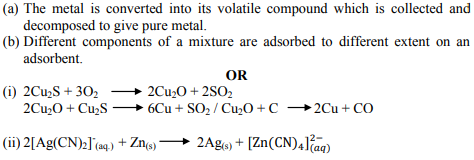Question 23: (Marks 2)

Write the balanced chemical equations involved in the preparation of KMnO4 from pyrolusite ore (MnO2).

OR

Write the balanced ionic equations showing the oxidising action of acidified dichromate (Cr2O2–7) solution with (i) Iron (II) Ion and (ii) tin (II) ion.

Answer :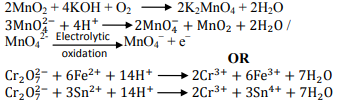Question 24: (Marks 2)

Write the IUPAC names and hybridisation of the following complexes :

(i) [Ni(CN)4]2–

(ii) [Fe(H2O)6]2+

(Given : Atomic number Ni = 28, Fe = 26)

Answer :

(i) Tetracyanidonickelate(II) / Tetracyanonickelate(II)

dsp2

(ii) Hexaaquairon(II)

sp3d2

Question 25: (Marks 2)

Define the following terms with a suitable example in each :

(i) Antibiotics

(ii) Antiseptics

Answer :

(i) A chemical substance which in low concentrations inhibits the growth or destroys microorganisms.

eg: Pencillin / Ofloxacin / Chloramphenicol / Tetracycline

(ii) Antiseptics are the chemical substances applied to the living tissues which prevent the growth or kill the microorganisms.

eg: Dettol / Furacine / Soframycine.

(or any other suitable example)

Question 26: (Marks 2)

Write the reactions showing the presence of following in the open structure of glucose :

(i) a carbonyl group

(ii) Straight chain with six carbon atoms

Answer :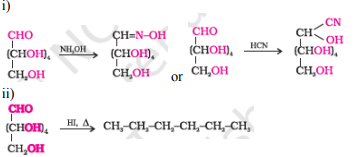Question 27: (Marks 2)

State Henry’s law. Calculate the solubility of CO2 in water at 298 K under 760 mm Hg.

(KH for CO2 in water at 298 K is 1.25 × 106 mm Hg)

Answer :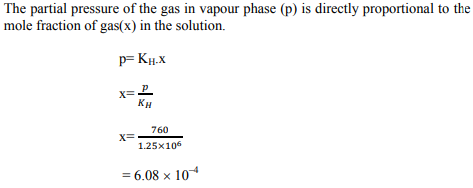SECTION – C

Question 28: (Marks 3)

The freezing point of a solution containing 5g of benzoic acid (M = 122 g mol–1) in 35g of benzene is depressed by 2.94 K. What is the percentage association of benzoic acid if it forms a dimer in solution ? (Kf for benzene = 4.9 K kg mol–1)

Answer :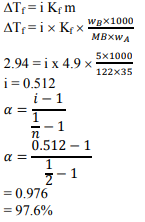Question 29: (Marks 3)

The rate constant for the first order decomposition of N2O5 is given by the following equation : k = (2.5 × 1014 s–1) e(–25000K)/T Calculate Ea for this reaction and rate constant if its half-life period be 300 minutes.

Answer :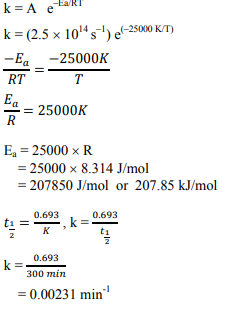Question 30: (Marks 3)

Write the name and structures of monomer(s) in the following polymers :

(i) Nylon-6

(ii) PVC

(iii) Neoprene

Answer :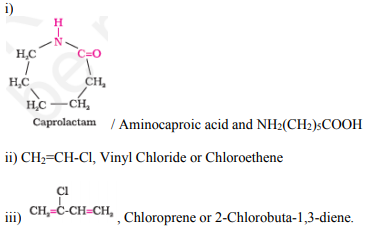Question 31: (Marks 3)

Following ions are given :

Cr2+, Cu2+, Cu+, Fe2+, Fe3+, Mn3+

Identify the ion which is

(i) a strong reducing agent.

(ii) unstable in aqueous solution.

(iii) a strong oxiding agent.

Give suitable reason in each.

Answer :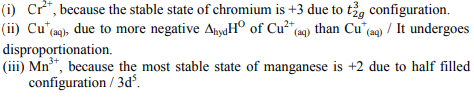Question 32: (Marks 3)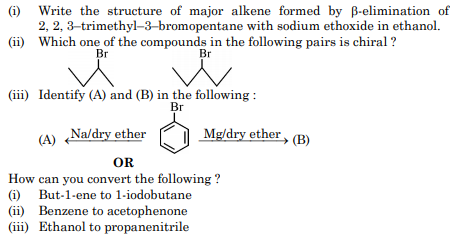Answer :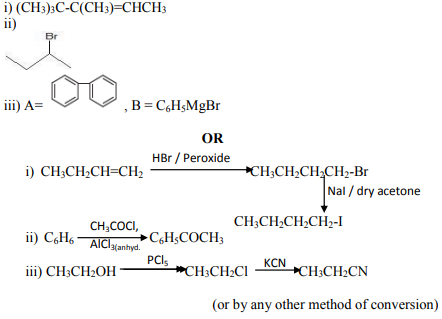Question 33: (Marks 3)

Arrange the following compounds as directed :

(i) In increasing order of solubility in water :

(CH3)2NH, CH3NH2, C6H5NH2

(ii) In decreasing order of basic strength in aqueous solution :

(CH3)3N, (CH3)2NH, CH3NH2

(iii) In increasing order of boiling point :

(C2H5)2NH, (C2H5)3N, C2H5NH2

Answer :

(i) C6H5NH2 < (CH3)2NH < CH3NH2

(ii) (CH3)2NH > CH3NH2 > (CH3)3N

(iii) (C2H5)3N < (C2H5)2NH < C2H5NH2

Question 34: (Marks 3)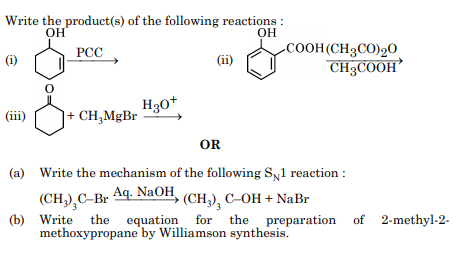Answer :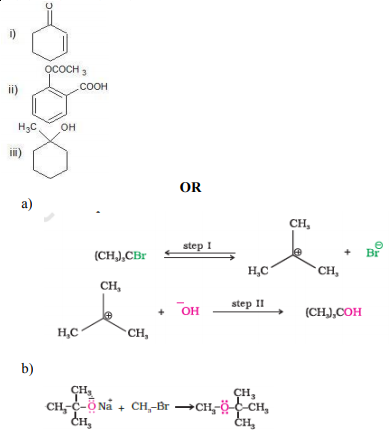SECTION – D

Question 35: (Marks 5)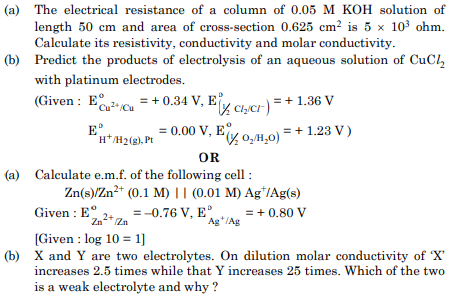Answer :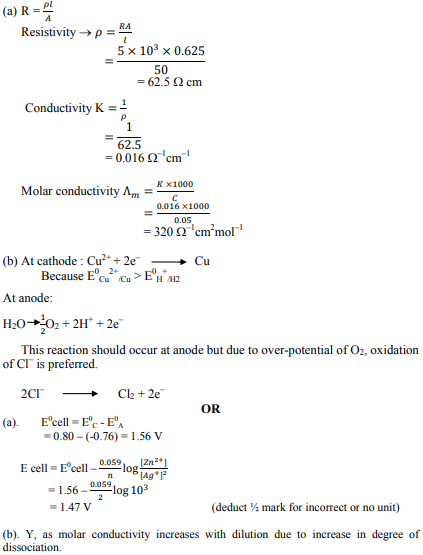Question 36: (Marks 5)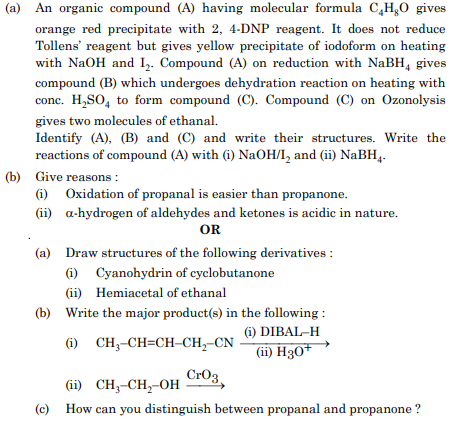Answer :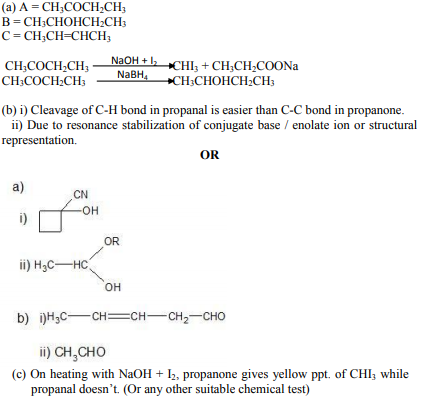Question 37: (Marks 5)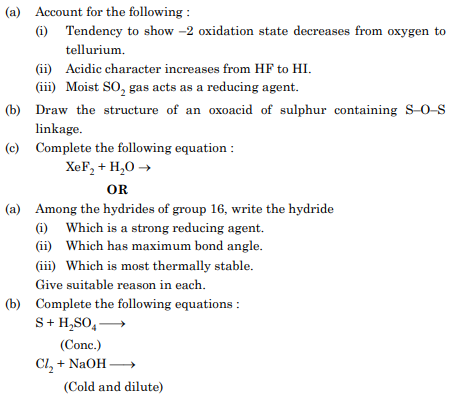Answer :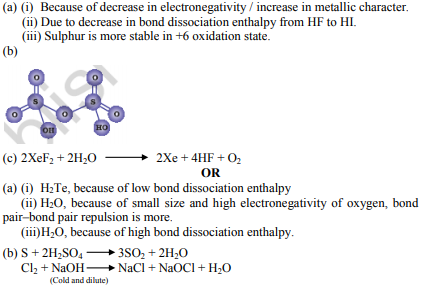☞ Click here for privious year Question papers

## Chemistry sample paper class 12, Chemistry previous year question paper class 12, cbse class 12 Chemistry sample paper, cbse class 12 Chemistry sample paper 2020, Chemistry sample paper class 12 2020, cbse sample paper 2020 class 12 Chemistry, class 12 Chemistry sample paper 2020, class 12 important questions Chemistry, cbse class 12 board exam Chemistry paper, Chemistry previous year question papers class 12 with solutions, Chemistry sample paper class 12 2019, cbse class 12 Chemistry question paper 2017 solved pdf, cbse class 12 Chemistry question paper 2018, class 12 Chemistry paper 2019, Chemistry question paper for class 12, cbse class 12 Chemistry paper 2019

### NCERT Books Free Pdf Download for Class 5, 6, 7, 8, 9, 10 , 11, 12 Hindi and English Medium

 Mathematics Biology Psychology Chemistry English Economics Sociology Hindi Business Studies Geography Science Political Science Statistics Physics Accountancy

## Please Share this webpage on facebook, whatsapp, linkdin and twitter.

Copyright @ ncerthelp.com A free educational website for CBSE, ICSE and UP board.